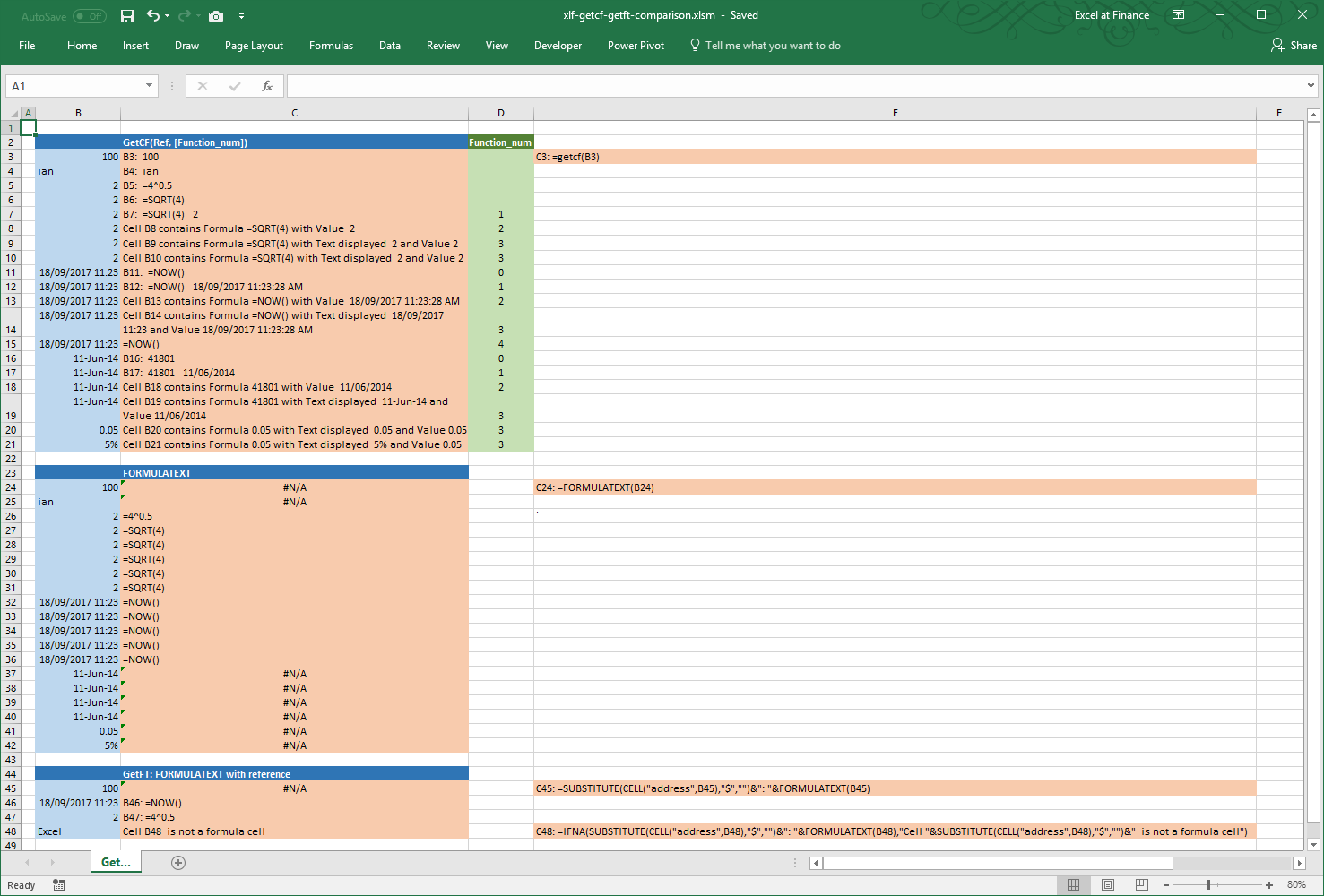# xlf | GetFT formula

## 1. GetFT - about the formula

Description: returns the formula or text of a relative reference with the address of the reference.

Syntax: GetFT.1L, GetFT.2L, GetFT.1U

Comments: GetFT is a custom version of the Excel FORMULATEXT function, introduced in Excel 2013. It is an alternative to the excelatfinance.com GetCF function in situations where the workbook is not macro enabled.

## 2. GetFT - setting up the formula in Excel

To setup the formulas manually, follow steps 1 to 12. See section 3 for VBA code to perform the setup.

Suppose that the active cell is E10, then add the following new relative offset names (items 1 to 6):

1. Name: OneLeft
2. RefersTo: =!D10

3. Name: TwoLeft
4. RefersTo: =!C10

5. Name: OneUp
6. RefersTo: =!E9

With the relative offsets in place, add the GetFT name variations (items 7 to 12):

1. Name: GetFT.1L
2. RefersTo: =IF(ISFORMULA(OneLeft),ADDRESS(ROW(OneLeft),COLUMN(OneLeft),4)&": "&FORMULATEXT(OneLeft),ADDRESS(ROW(OneLeft),COLUMN(OneLeft),4)&": "&IF(NOT(ISBLANK(OneLeft)),OneLeft,""))

3. Name: GetFT.2L
4. RefersTo: =IF(ISFORMULA(TwoLeft),ADDRESS(ROW(TwoLeft),COLUMN(TwoLeft),4)&": "&FORMULATEXT(TwoLeft),ADDRESS(ROW(TwoLeft),COLUMN(TwoLeft),4)&": "&IF(NOT(ISBLANK(TwoLeft)),TwoLeft,""))

5. Name: GetFT.1U
6. RefersTo: =IF(ISFORMULA(OneUp),ADDRESS(ROW(OneUp),COLUMN(OneUp),4)&": "&FORMULATEXT(OneUp),ADDRESS(ROW(OneUp),COLUMN(OneUp),4)&": "&IF(NOT(ISBLANK(OneUp)),OneUp,""))

Items 8, 10, and 12 are each a one line statement, and contain similar syntax.

### Discussion

GetCF.xx contains 3 logical tests.

Looking at the GetCF.1L example in cell C3 of the Excel Web App #1 in figure 1, the reference cell B3, is one cell left of the GetCF formula cell, requiring GetCF.1L

Selected Excel functions used in this module.

Excel functions Description
ADDRESS(row_num, column_num, [abs_num], [a1], [sheet_text])Returns the address of a cell from specified row and column numbers
[abs_num]: 1 (default) is absolute; 2 is absolute row, relative column; 3 is relative row, absolute column, and 4 is relative
[a1]: TRUE (default) returns A1-style, and FALSE returns R1C1 reference style
[sheet_text] is the name of the worksheet
FORMULATEXT (reference)Returns a formula as a string
ISFORMULA(reference)
New in Excel 2013
Returns a Boolean TRUE when the cell contains a formula and FALSE if not

### GetFT generic:

1. Target cell: B46: =NOW()
2. Formula: =SUBSTITUTE(CELL("address",B46),"$","")&": "&FORMULATEXT(B46) returns B46: =NOW() 3. Target cell: B48: Excel 4. Formula: =IFNA(SUBSTITUTE(CELL("address",B48),"$","")&": "&FORMULATEXT(B48),"Cell "&SUBSTITUTE(CELL("address",B48),"\$","")&" is not a formula cell") returns Cell B48 is not a formula cellFig 2: GetCF and GetFT comparison #2 - download the file xlf-getcf-getft-comparison.xlsm [19 KB]

## 3 GetFT - the VBA code

Setup the formulas with the SetNames procedure in code 1

Code 1: Macro SetNames to add the relative addresses and formulas to the Name Manager list
Private Sub SetNames()
'
' Requires Function DNameExist
'
Dim NameList(1 To 6, 1 To 3) As String
Dim i As Integer, j As Integer

NameList(1, 1) = "OneLeft"
NameList(1, 2) = "=!RC[-1]"
NameList(1, 3) = "Cell one column left (relative)"

NameList(2, 1) = "TwoLeft"
NameList(2, 2) = "=!RC[-2]"
NameList(2, 3) = "Cell two columns left (relative)"

NameList(3, 1) = "OneUp"
NameList(3, 2) = "=!R[-1]C"
NameList(3, 3) = "Cell one row above (relative)"

NameList(4, 1) = "GetFT.1L"
NameList(4, 2) = "=IF(ISFORMULA(OneLeft),ADDRESS(ROW(OneLeft),COLUMN(OneLeft),4)&"": ""&FORMULATEXT(OneLeft),ADDRESS(ROW(OneLeft),COLUMN(OneLeft),4)&"": ""&IF(NOT(ISBLANK(OneLeft)),OneLeft,""""))"
NameList(4, 3) = "FormulaText one left"

NameList(5, 1) = "GetFT.2L"
NameList(5, 2) = "=IF(ISFORMULA(TwoLeft),ADDRESS(ROW(TwoLeft),COLUMN(TwoLeft),4)&"": ""&FORMULATEXT(TwoLeft),ADDRESS(ROW(TwoLeft),COLUMN(TwoLeft),4)&"": ""&IF(NOT(ISBLANK(TwoLeft)),TwoLeft,""""))"
NameList(5, 3) = "FormulaText two left"

NameList(6, 1) = "GetFT.1U"
NameList(6, 2) = "=IF(ISFORMULA(OneUp),ADDRESS(ROW(OneUp),COLUMN(OneUp),4)&"": ""&FORMULATEXT(OneUp),ADDRESS(ROW(OneUp),COLUMN(OneUp),4)&"": ""&IF(NOT(ISBLANK(OneUp)),OneUp,""""))"
NameList(6, 3) = "FormulaText one up"

For i = 1 To UBound(NameList, 1)
If DNameExist(NameList(i, 1)) = False Then
Names.Add Name:=NameList(i, 1), _
RefersToR1C1:=NameList(i, 2)
Names(NameList(i, 1)).Comment = NameList(i, 3)
' Names(NameList(i, 1)).Visible = False
End If
Next i

End Sub


About Code 1

1. Lines 8 to 10: assign values of the Name object to elements of a string array.
Line 8 - Name:
Line 9 - Refers to:
Line 10 - Comment:
The assignment statements are repeated, to allow use of the For...Next loop in lines 32 to 39
2. Lines 34 to 36 code statements of the For...Next loop used to set the properties of the Names object Add method
Line 34 - Name:= (from line 10
Line 35 - RefersToR1C:= (from line 11)
Line 36 - Comment (from line 10)

If the name already exists, then the Names.Add method in code 1, lines 34 to 35 will return an error. To trap this error, the DNameExists function is implemented (code 2).

## 3.2 DNameExist - the VBA code

Code 2: Function DNameExist returns a Boolean TRUE if the string DName is a defined name in the active workbook
Private Function DNameExist(DName As String) As Boolean
Dim TestName As Name

On Error Resume Next
Set TestName = Names(DName)
If TestName Is Nothing Then
DNameExist = False
Else
DNameExist = True
End If
On Error GoTo 0

End Function


• This example was developed in Excel 2016 64 bit.
• Published: 14 April 2016
• Revised: Tuesday 11th of June 2019 - 10:41 PM, Pacific Time (PT)